# Common Core: High School - Geometry : Transformation and Congruence of Rigid Motions: CCSS.Math.Content.HSG-CO.B.6

## Example Questions

← Previous 1

### Example Question #1 : Transformation And Congruence Of Rigid Motions: Ccss.Math.Content.Hsg Co.B.6

Determine whether the statement is true or false.

For a translation to be considered rigid, the starting and ending figures must be congruent.

True

False

True

Explanation:

Recall that a rigid motion is that that preserves the distances while undergoing a motion in the plane. This is also called an isometry, rigid transformations, or congruence transformations and there are four different types. Therefore, for the translation to be considered "rigid" the two figures must be congruent by definition of a rigid motion.

Therefore, the statement, "For a translation to be considered rigid, the starting and ending figures must be congruent." is true.

### Example Question #2 : Transformation And Congruence Of Rigid Motions: Ccss.Math.Content.Hsg Co.B.6

Which of the following is NOT a rigid motion?

Rotation

Translation

Expansion

Reflection

Glide Reflection

Expansion

Explanation:

Recall that a rigid motion is that that preserves the distances while undergoing a motion in the plane. This is also called an isometry, rigid transformations, or congruence transformations and there are four different types.

These basic type of rigid motions include the following:

1. Rotation
2. Reflection
3. Translation
4. Glide Reflection

Therefore, of the answer selections, "Expansion" is the term that is NOT a rigid motion.

### Example Question #1 : Transformation And Congruence Of Rigid Motions: Ccss.Math.Content.Hsg Co.B.6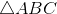has a rigid motion applied to it that results in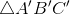. What can be concluded about the relationship between these two triangles.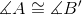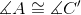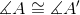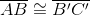Explanation:

A rigid motion is that that preserves the distances while undergoing a motion in the plane. This is also called an isometry, rigid transformations, or congruence transformations and there are four different types.

Regardless of which type of rigid motion occurred, the following is true about the triangles' angles: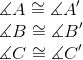The following is also true about the side lengths: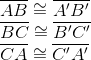Therefore, of the answer choicesis the correct choice.

### Example Question #1 : Transformation And Congruence Of Rigid Motions: Ccss.Math.Content.Hsg Co.B.6has a rigid motion applied to it that results in. What can be concluded about the relationship between these two triangles.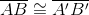Explanation:

A rigid motion is that that preserves the distances while undergoing a motion in the plane. This is also called an isometry, rigid transformations, or congruence transformations and there are four different types.

Regardless of which type of rigid motion occurred, the following is true about the triangles' angles:The following is also true about the side lengths:Therefore, of the answer choicesis the correct choice.

### Example Question #1 : Transformation And Congruence Of Rigid Motions: Ccss.Math.Content.Hsg Co.B.6

Determine whether the statement is true or false:

A glide reflection is a synonym for a rigid motion translation.

False

True

False

Explanation:

A glide reflection is a rigid motion that occurs when a figure is translated a certain distance and then reflected or reflected and then translated.

A translation is a rigid motion describing when a object is moved a certain distance.

A glide reflection contains a translation but it is not a synonym for translation therefore, the statement is false.

### Example Question #1 : Transformation And Congruence Of Rigid Motions: Ccss.Math.Content.Hsg Co.B.6

Determine whether the statement is true or false:

All glide reflections are reflections.

False

True

True

Explanation:

A glide reflection is a rigid motion that occurs when a figure is translated a certain distance and then reflected or reflected and then translated. In either case, a glide reflection aways contains a reflection.

Therefore, the statement, "All glide reflections are reflections." is true.

### Example Question #52 : Congruence

Sally has a quarter that is face up on the desk. If she slides it to Bob on the other side of the desk and he flips it over so that the tails side is facing up, is it a rigid motion?

No

Yes

Yes

Explanation:

In the situation where Sally has a quarter that is face up on the desk. The coin is the object. Then she slides it to Bob on the other side of the desk and he flips it over so that the tails side is facing up. Therefore, since the coin maintains it shape it is undergoing a translation to reach the other side of the desk and a reflection to flip the coin. This describes a glide reflection which is in fact, a rigid motion.

Therefore, the statement is describing a rigid motion. The answer is "Yes"

### Example Question #53 : Congruence

Sally has a quarter that is face up on the desk. Then she slides the coin to Bob on the other side of the desk and he flips it over so that the tails side is facing up. What type of rigid motion does this situation describe?

Reflection

Rotation

Glide Reflection

Translation

This is not a rigid motion.

Glide Reflection

Explanation:

In the situation where Sally has a quarter that is face up on the desk. The coin is the object. Then she slides it to Bob on the other side of the desk and he flips it over so that the tails side is facing up. Therefore, since the coin maintains it shape it is undergoing a translation to reach the other side of the desk and a reflection to flip the coin. This describes a glide reflection which is in fact, a rigid motion.

### Example Question #54 : Congruence

Jane and Bob are filling up water balloons for a party they are throwing. Jane thinks the balloons should have more water in them so she fills them fuller. Each water balloon's circumference is one inch greater than before. Does this describe a rigid motion?

No

Yes

No

Explanation:

Recall that a rigid motion is that that preserves the distances between points within the object while undergoing a motion in the plane. This is also called an isometry, rigid transformations, or congruence transformations and there are four different types. The water balloons are the objects in this situation. Since the they are filled with more water to increase their circumferences, it does not preserve the shape of the object and thus, is not a rigid motion.

### Example Question #70 : Congruence

Select the answer that best completes the following definition:

A motion that preserves distance in the plane is called a __________

Non-Rigid Motion

Transformation

Rigid Motion

Rotation

Reflection

Rigid Motion

Explanation:

A rigid motion is that that preserves the distances while undergoing a motion in the plane. This is also called an isometry, rigid transformations, or congruence transformations and there are four different types.

These basic type of rigid motions include the following:

1. Rotation
2. Reflection
3. Translation
4. Glide Reflection

Therefore, "A motion that preserves distance in the plane is called a rigid motion".

← Previous 1

### All Common Core: High School - Geometry Resources